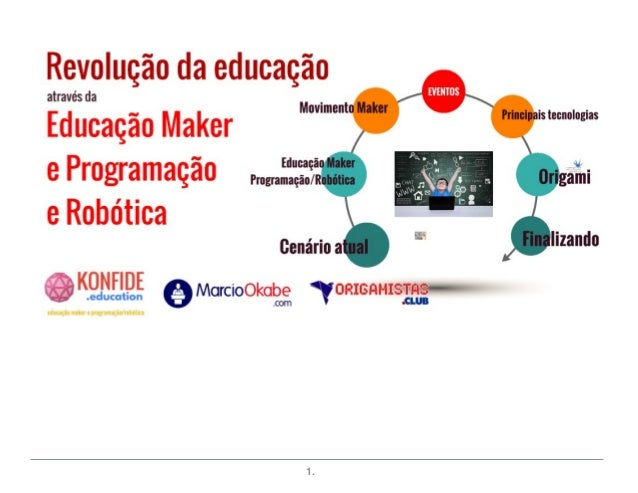Successfully reported this slideshow.Upcoming SlideShare
×

Revolução da Educação - Movimento Maker e Programação/Robótica

122 views

Published on

Palestra sobre Revolução da Educação através do Movimento Maker e Programação/Robótica

Published in: Education
• Full Name
Comment goes here.

Are you sure you want to Yes No• Be the first to comment

• Be the first to like this

1. 1. 1.
2. 2. 2.
3. 3. 3.
4. 4. 4.
5. 5. 5.
6. 6. 6.
7. 7. 7.
8. 8. 8.
9. 9. 9.
10. 10. 10.
11. 11. 11.
12. 12. 12.
13. 13. 13.
14. 14. 14.
15. 15. 15.
16. 16. 16.
17. 17. 17.
18. 18. 18.
19. 19. 19.
20. 20. 20.
21. 21. 21.
22. 22. 22.
23. 23. 23.
24. 24. 24.
25. 25. 25.
26. 26. 26.
27. 27. 27.
28. 28. 28.
29. 29. 29.
30. 30. 30.
31. 31. 31.
32. 32. 32.
33. 33. 33.
34. 34. 34.
35. 35. 35.
36. 36. 36.
37. 37. 37.
38. 38. 38.
39. 39. 39.
40. 40. 40.
41. 41. 41.
42. 42. 42.
43. 43. 43.
44. 44. 44.
45. 45. 45.
46. 46. 46.
47. 47. 47.
48. 48. 48.
49. 49. 49.
50. 50. 50.
51. 51. 51.
52. 52. 52.
53. 53. 53.
54. 54. 54.
55. 55. 55.
56. 56. 56.
57. 57. 57.
58. 58. 58.
59. 59. 59.
60. 60. 60.
61. 61. 61.
62. 62. 62.
63. 63. 63.
64. 64. 64.
65. 65. 65.
66. 66. 66.
67. 67. 67.
68. 68. 68.
69. 69. 69.
70. 70. 70.
71. 71. 71.
72. 72. 72.
73. 73. 73.
74. 74. 74.
75. 75. 75.
76. 76. 76.
77. 77. 77.
78. 78. 78.
79. 79. 79.
80. 80. 80.
81. 81. 81.
82. 82. 82.
83. 83. 83.
84. 84. 84.
85. 85. 85.
86. 86. 86.
87. 87. 87.
88. 88. 88.
89. 89. 89.
90. 90. 90.
91. 91. 91.
92. 92. 92.
93. 93. 93.
94. 94. 94.
95. 95. 95.
96. 96. 96.